### Fitting Algorithms

The multivariate adaptive regression splines algorithm (Friedman, 1991b) is a predictive modeling algorithm that combines nonparametric variable transformations with a recursive partitioning scheme.

The algorithm originates with Smith (1982), who proposes a nonparametric method that applies the model selection method (stepwise regression) to a large number of truncated power spline functions, which are evaluated at different knot values. This method constructs spline functions and selects relevant knot values automatically with the model selection method. However, the method is applicable only to problems in low dimensions. For multiple variables, the number of tensor products between spline basis functions is too large to fit even a single model. The multivariate adaptive regression splines algorithm avoids this situation by using forward selection to build the model gradually instead of using the full set of tensor products of spline basis functions.

Like the recursive partitioning algorithm, which has growing and pruning steps, the multivariate adaptive regression splines algorithm contains two stages: forward selection and backward selection. During the forward selection process, bases are created from interactions between existing parent bases and nonparametric transformations of continuous or classification variables as candidate effects. After the model grows to a certain size, the backward selection process begins by deleting selected bases. The deletion continues until the null model is reached, and then an overall the best model is chosen based on some goodness-of-fit criterion. The next three subsections give details about the selection process and methods of nonparametric transformation of variables. The fourth subsection describes how the multivariate adaptive regression splines algorithm is applied to fit generalized linear models. The fifth subsection describes the fast algorithm (Friedman, 1993) for speeding up the fitting process.

#### Forward Selection

The forward selection process in the multivariate adaptive regression splines algorithm is as follows:

1. Initialize by setting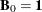and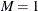.

2. Repeat the following steps until the maximum number of bases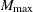has been reached or the model cannot be improved by any combination of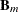,, and t.

1. Set the lack-of-fit criterion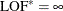.

2. For each selected basis: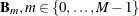do the following for each variablethatdoes not consist of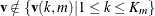1. For each knot value (or a subset of categories) t of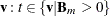, form a model with all currently selected bases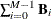and two new bases: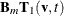and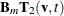.

2. Compute the lack-of-fit criterion for the new model LOF.

3. If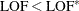, then update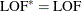,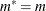,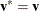, and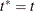.

3. Update the model by adding two bases that improve the most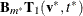and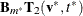.

4. Set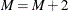.

The essential part of each iteration is to search a combination of,, and t such that adding two corresponding bases most improve the model. The objective of the forward selection step is to build a model that overfits the data. The lack-of-fit criterion for linear models is usually the residual sum of squares (RSS).

#### Backward Selection

The backward selection process in the multivariate adaptive regression splines algorithm is as follows:

1. Initialize by setting the overall lack-of-fit criterion:.

2. Repeat the following steps until the null model is reached. The final model is the best one that is found during the backward deletion process.

1. For a selected basis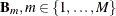:

1. Compute the lack-of-fit criterion, LOF, for a model that excludes.

2. If, save the model as the best one. Let.

3. Delete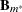from the current model.

2. Set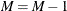.

The objective of the backward selection is to prune back the overfitted model to find the best model that has good predictive performance. So the lack-of-fit criteria that characterize model loyalty to original data are not appropriate. Instead, the multivariate adaptive regression splines algorithm uses a quantity similar to the generalized cross validation criterion. See the section Goodness-of-Fit Criteria for more information.

#### Variable Transformations

The type of transformation depends on the variable type:

• For a continuous variable, the transformation is a linear truncated power spline,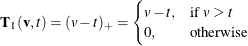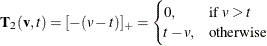where t is a knot value for variableand v is an observed value for. Instead of examining every unique value of, a series of knot values with a minimum span are used by assuming the smoothness of the underlying function. Friedman (1991b) uses the following formula to determine a reasonable number of counts between knots (span size). For interior knots, the span size is determined by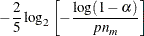For boundary knots, the span size is determined by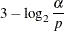whereis the parameter that controls the knot density, p is the number of variables, and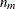is the number of observations that a parent basis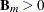.

• For a classification variable, the transformation is an indicator function,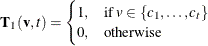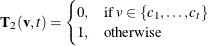where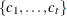is a subset of all categories of variable. The smoothing is applied to categorical variables by assuming that subsets of categories tend to have similar properties, analogous to the assumption that a local neighborhood has close predictions for continuous variables.

If a categorical variable has k distinct categories, then there are a total of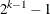possible subsets to consider. The computation cost is equal to all-subsets selection in regression, which is expensive for large k values. The multivariate adaptive regression splines algorithm use the stepwise selection method to select categories to form the subset. The method is still greedy, but it reduces computation and still yields reasonable final models.

#### Goodness-of-Fit Criteria

Like other nonparametric regression procedures, the multivariate adaptive regression splines algorithm can yield complicated models that involve high-order interactions in which many knot values or subsets are considered. Besides the basis functions, both the forward selection and backward selection processes are also highly nonlinear. Because of the trade-off between bias and variance, the complicated models that contain many parameters tend to have low bias but high variance. To select models that achieve good prediction performance, Craven and Wahba (1979) propose the widely used generalized cross validation criterion (GCV),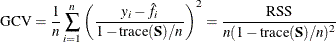where y is the response,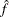is an estimate of the underlying smooth function, and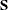is the smoothing matrix such that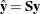. The effective degrees of freedom for the smoothing spline can be defined as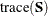. In the multivariate adaptive regression splines algorithm, Friedman (1991b) uses a similar quantity as the lack-of-fit criterion,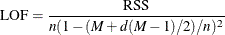where d is the degrees-of-freedom cost for each nonlinear basis function and M is total number of linearly independent bases in the model. Because any candidate model that is evaluated at each step of the multivariate adaptive regression splines algorithm is a linear model, M is actually the trace of the hat matrix. The only difference between the GCV criterion and the LOF criterion is the extra term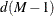. The corresponding effective degrees of freedom is defined as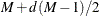. The quantity d takes into account the extra nonlinearity in forming new bases, and it operates as a smoothing parameter. Larger values of d tend to result in smoother function estimates. Based on many practical experiments and some theoretic work (Owen, 1991), Friedman suggests that the value of d is typically in the range of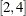. For data that have complicated structures, the value of d could be much larger.

Alternatively, you can use the cross validation as the goodness-of-fit criterion or use a separate validation data set to select models and a separate testing data set to evaluate selected models.

#### Generalized Linear Models

Friedman (1991b) applies the multivariate adaptive regression splines algorithm to a logistic model by using the squared error loss between the response and inversely linked values in the goodness-of-fit criterion: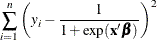When a final model is obtained, the ordinary logistic model is fitted on selected bases. Some realizations of the multivariate adaptive regression splines algorithm ignore the distributional properties and derive model bases that are based on the least squares criterion. The reason to ignore the distributional properties or use least squares approximations is that examining the lack-of-fit criterion for each combination of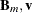, and t is computationally formidable, because one generalized linear model fit involves multiple steps of weighted least squares. The ADAPTIVEREG procedure extends the multivariate adaptive regression splines algorithm to generalized linear models as suggested by Buja et al. (1991).

In the forward selection process, the ADAPTIVEREG procedure extends the algorithm in the following way. Suppose there are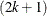bases after the kth iteration. Then a generalized linear model is fitted against the data by using the selected bases. Then the weighted least squares method uses the working weights and working response in the last step of the iterative reweighted least squares algorithm as the weight and response for selecting new bases in the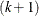th iteration. Then the residual chi-square statistic is used to select two new bases. This is similar to the forward selection scheme that the LOGISTIC procedure uses. For more information about the score chi-square statistic, see the section Testing Individual Effects Not in the Model in Chapter 54: The LOGISTIC Procedure.

In the backward selection process, the ADAPTIVEREG procedure extends the algorithm in the following way. Suppose there are M bases in the selected model. The Wald chi-square statistic is used to determine which basis to delete. After one basis is selected for deletion, a generalized linear model is refitted with the remaining bases. This is similar to the backward deletion scheme that the LOGISTIC procedure uses. For more information about the Wald chi-square statistic, see the section Testing Linear Hypotheses about the Regression Coefficients in Chapter 54: The LOGISTIC Procedure.

Accordingly, the lack-of-fit criterion in the forward selection for generalized linear models is the score chi-square statistic. For the lack-of-fit criterion in the backward selection process for generalized linear models, the residual sum of squares term is replaced by the model deviance.

#### Fast Algorithm

The original multivariate adaptive regression splines algorithm is computationally expensive. To improve the computation speed, Friedman (1993) proposes the fast algorithm. The essential idea of the fast algorithm is to reduce the number of combinations of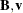, and t that are examined at each step of forward selection.

Suppose there arebases that are formed after the kth iteration, where a parent basisis selected to construct two new bases. Consider a queue with bases as its elements. At the top of the queue are the two newly constructed bases,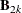and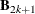. The rest of the queue is sorted based on the minimum lack-of-fit criterion for each basis: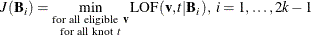When k is not small, there are a relatively large number of bases in the model, and adding more bases is unlikely to dramatically improve the fit. Thus the ranking of the bases in the priority queue is not likely to change much during adjacent iterations. So the candidate parent bases can be restricted to the top K ones in the queue forth iteration. After the kth iteration, the top bases have new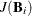values, whereas the values of the bottom bases are unchanged. The queue is reordered based onvalues. This corresponds to the K= option value for the FAST option in the MODEL statement.

To avoid losing the candidate bases that are ranked at the bottom of the queue and to allow them to rise back to the top, a natural aging factor is introduced into each basis. This is accomplished by defining the priority for each basis function to bewhere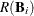is the rank of ith basis in the queue,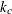is the current iteration number, and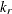is the number of the iteration where thevalue was last computed. The top K candidate bases are then sorted again based on this priority. Large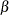values cause bases that have low improvement during previous iterations to rise faster to the top of the list. This corresponds to the BETA= value for the FAST option in the MODEL statement.

For a candidate basis in the top of the priority queue, the minimum lack-of-fit criterionis recomputed for all eligible variablesfor theiteration. An optimal variable is likely to be the same as the one that was found during the previous iteration. So the fast multivariate adaptive regression splines algorithm introduces another factor H to save the computation cost. The factor specifies how oftenshould be recomputed for all eligible variables. If H = 1, then optimization over all variables is done at each iteration when a parent basis is considered. If H = 5, the complete optimization is done after five iterations. For an iteration count less than the specified H, the optimization is done only for the optimal variable found in the last complete optimization. The only exceptions are the top three candidates,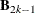(which is the parent basisused to construct two new bases) and two new ones,and. The complete optimization for them is performed at each iteration. This corresponds to the H= option value for the FAST option in the MODEL statement.